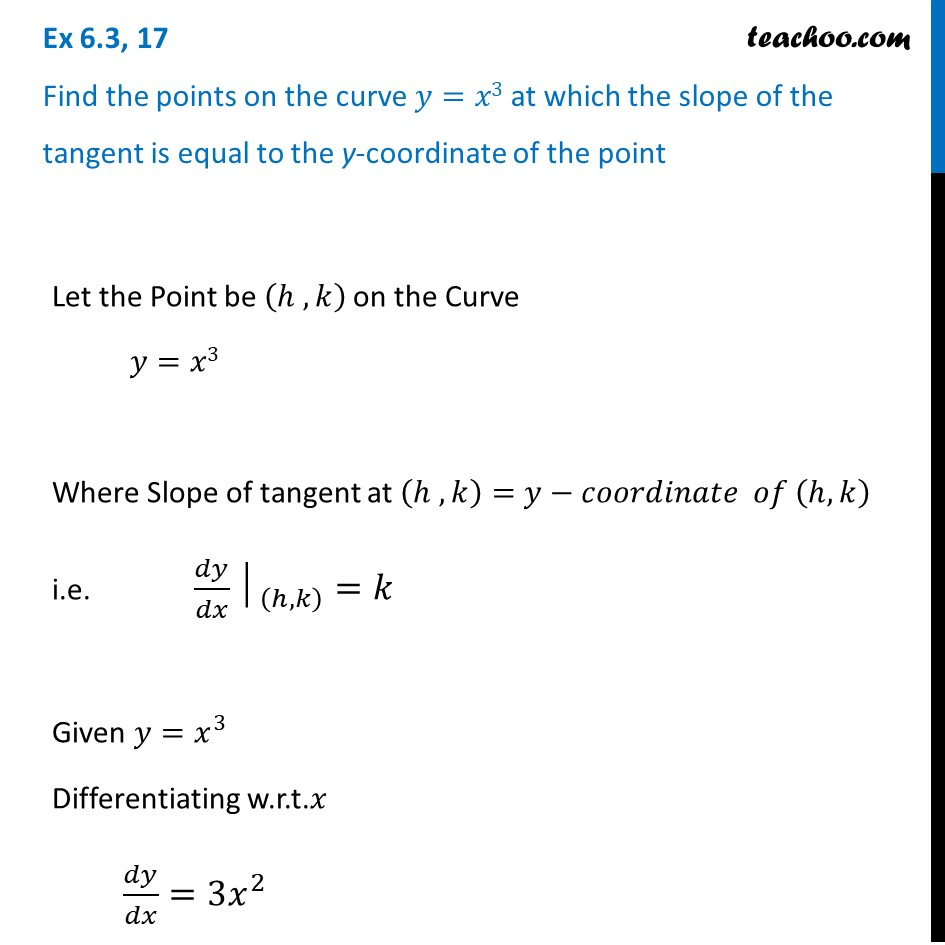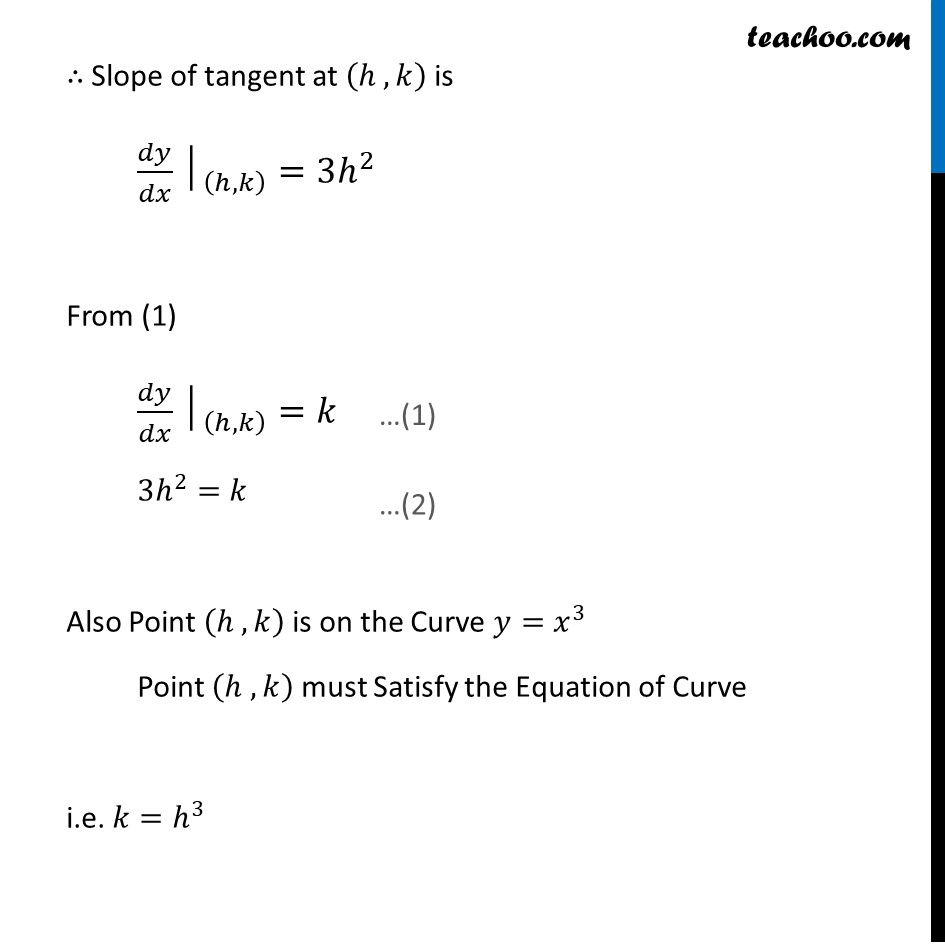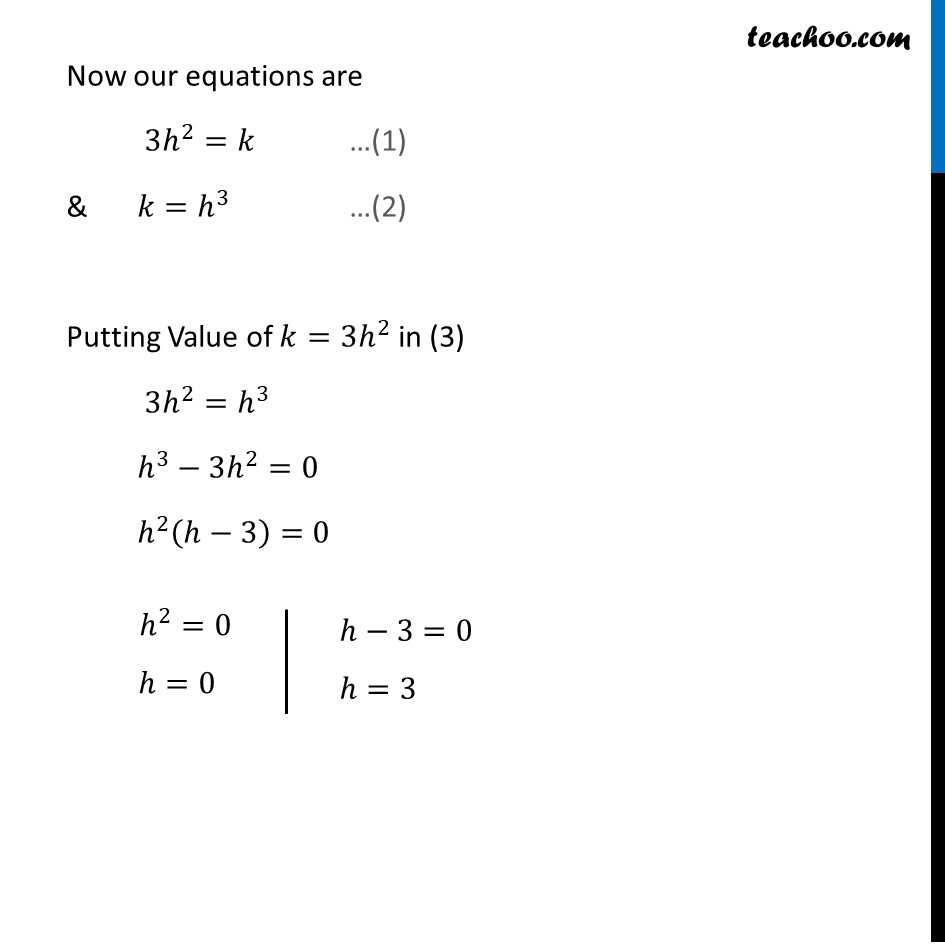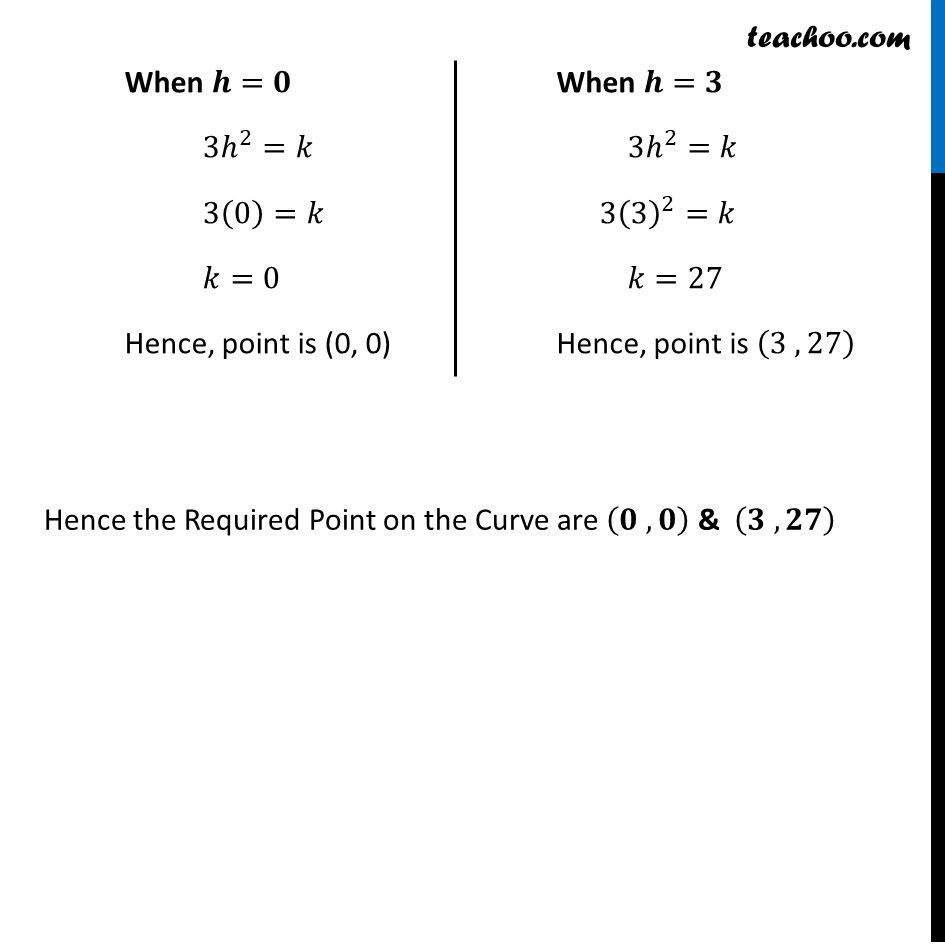Ex 6.3

Chapter 6 Class 12 Application of Derivatives
Serial order wiseGet live Maths 1-on-1 Classs - Class 6 to 12

### Transcript

Ex 6.3, 17 Find the points on the curve 𝑦=𝑥3 at which the slope of the tangent is equal to the y-coordinate of the pointLet the Point be (ℎ , 𝑘) on the Curve 𝑦=𝑥3 Where Slope of tangent at (ℎ , 𝑘)=𝑦−𝑐𝑜𝑜𝑟𝑑𝑖𝑛𝑎𝑡𝑒 𝑜𝑓 (ℎ, 𝑘) i.e. 〖𝑑𝑦/𝑑𝑥│〗_((ℎ, 𝑘) )=𝑘 Given 𝑦=𝑥^3 Differentiating w.r.t.𝑥 𝑑𝑦/𝑑𝑥=3𝑥^2 ∴ Slope of tangent at (ℎ , 𝑘) is 〖𝑑𝑦/𝑑𝑥│〗_((ℎ, 𝑘) )=3ℎ^2 From (1) 〖𝑑𝑦/𝑑𝑥│〗_((ℎ, 𝑘) )=𝑘 3ℎ^2=𝑘 Also Point (ℎ , 𝑘) is on the Curve 𝑦=𝑥^3 Point (ℎ , 𝑘) must Satisfy the Equation of Curve i.e. 𝑘=ℎ^3 Now our equations are 3ℎ^2=𝑘 …(1) & 𝑘=ℎ^3 …(2) Putting Value of 𝑘=3ℎ^2 in (3) 3ℎ^2=ℎ^3 ℎ^3−3ℎ^2=0 ℎ^2 (ℎ−3)=0 ℎ^2=0 ℎ=0 ℎ−3=0 ℎ=3 When 𝒉=𝟎 3ℎ^2=𝑘 3(0)=𝑘 𝑘=0 Hence, point is (0, 0) When 𝒉=𝟑 3ℎ^2=𝑘 3(3)^2=𝑘 𝑘=27 Hence, point is (3 , 27)